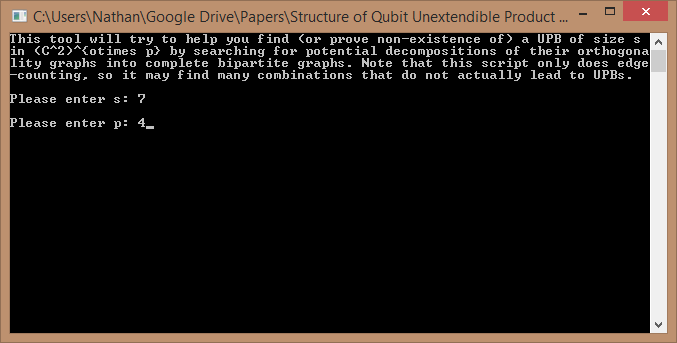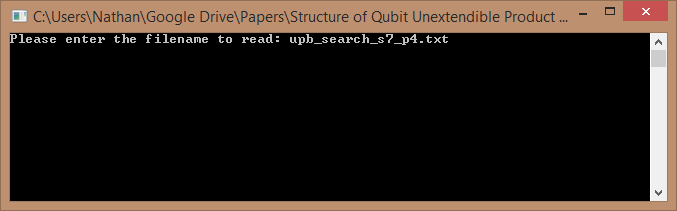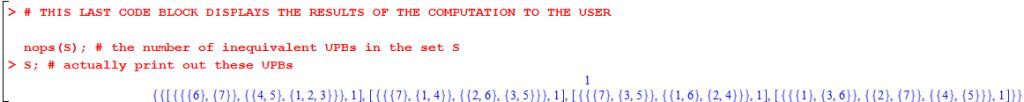## Code for Finding Qubit UPBs

This page contains C and Maple code that is used to construct the 4, 5, and 6-qubit UPBs found in  (and in particular, completely characterize 4-qubit UPBs and completely characterize qubit UPBs with 8 or fewer states).

Step 2: Unzip QUPB_search.zip (4 files should be unzipped: QUPB_check_decomp.c, QUPB_find_decomp.c, QUPB_remove_equivalent.mws, and QUPB_remove_equivalent.txt).

Step 3: Compile the two C scripts QUPB_check_decomp.c and QUPB_find_decomp.c (if you don’t know if you have a C compiler or how to install a compiler, it may be easiest to download and install CodeBlocks).

### Usage

This section will guide you through finding all 4-qubit UPBs of size 7 for illustrative purposes. It should then be clear how to find UPBs of different sizes on different numbers of qubits.

Step 1: Use QUPB_find_decomp.c

The script QUPB_find_decomp.c implements the search described in Step 1 of Appendix A of  — it finds complete bipartite graph decompositions that could possibly arise from the orthogonality graphs of UPBs. After compiling QUPB_find_decomp.c, run it:This creates the file upb_search_s7_p4.txt, which contains 24 possible decompositions of orthogonality graphs of 4-qubit UPBs on 7 states. For example, the first five lines of the file tell us that there might be such a UPB where the orthogonality graphs of the first 3 qubits are of the form$K_{2,2}\cup K_{2,1}$ and the orthogonality graph of the 4th qubit is of the form$K_{2,1}\cup K_{1,1}\cup K_{1,1}$. This file is used in Step 2 (below).

Step 2: Use QUPB_check_decomp.c

The script QUPB_check_decomp.c implements the brute-force search described in Step 2 of Appendix A of . That is, it reads in the output file from Step 1 (above) and finds all UPBs with the corresponding bipartite graph decompositions. After compiling QUPB_check_decomp.c, run it and enter the name of the file produced in Step 1:This creates the file results_upb_search_s7_p4.txt, which contains on each line (in a slightly weird Maple-readable format) a list of all UPBs corresponding to the graph decompositions in the input file. In this case, 4 UPBs were found. Some important notes:

• The UPBs that are found are not guaranteed to be inequivalent! That’s why Step 3 exists (below).
• If this part of the search takes too long, you can split the input text file (upb_search_s7_p4.txt) into two (or more) separate text files and run multiple instances of QUPB_check_decomp.c in parallel (if you have a multi-core CPU, each instance will run on a separate core).

Step 3: Use QUPB_remove_equivalent.mws

QUPB_remove_equivalent.mws is a Maple 16 worksheet that removes any UPBs in results_upb_search_s7_p4.txt that are equivalent to each other, leaving only a maximal set of inequivalent UPBs behind. A plaintext version of this Maple worksheet, which can be copy/pasted into any version of Maple, is also provided as QUPB_remove_equivalent.txt.

After specifying the value of s (i.e., the number of states in the UPBs: s = 7 in the example provided here) and copying the contents of results_upb_search_s7_p4.txt into the set S at the top of the Maple worksheet, running the worksheet results in this output (click on the image to enlarge):The blue “1” at the top of the output tells us that all 4 of the input UPBs were equivalent (i.e., there was only one inequivalent UPB). The line below that actually lists the UPB itself in a format that is moderately human-readable (on the first qubit, states 6 and 7 are orthogonal, and states 4 and 5 are orthogonal to states 1, 2, and 3, and so on for the other three qubits). We have thus shown that there is only one 7-state UPB on 4 qubits.

Update [May 5, 2014]: The scripts on this page were updated today, thanks to a bug report and improvements suggested by Vincent Russo.

### References

1. N. Johnston. The structure of qubit unextendible product bases. Journal of Physics A: Mathematical and Theoretical, 47:424034, 2014.